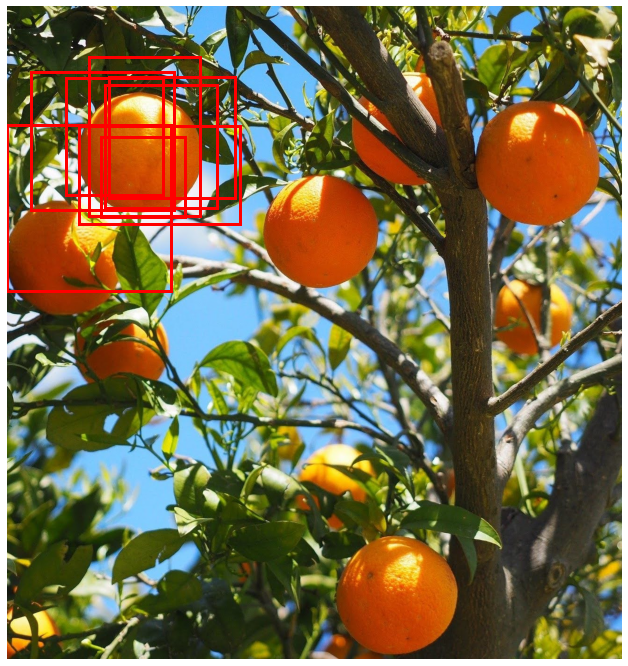Supporting batched data is an important requirement for a deep learning pipeline to enable time efficient model training. Often when writing a function, the easiest way to start is to first handle one element and then use a for loop over all the elements in the batch. With large datasets it is important to vectorize the code to enable a function to run in parallel on a batch of data instead of a single example at a time.

To demonstrate how to do this, let’s take the problem of calculating bounding box IOU. In an earlier post, we discussed ways of definining bounding boxes for object detection. In this post, we will consider the task of calculating the intersection over union between two potentially overlapping bounding boxes.

## Data

!curl -o oranges.jpg https://lh3.googleusercontent.com/pw/ACtC-3fLFL_y58xx1GVy6jLQ0quLpoctt-WG5yo5dR1N3RurI4Qodnnj_JeCEQG-kzILCAUNgZmcA5QlkuLYnbW33Y1XTj48knehvFywJoz1ni3U6MtGiiJzvz4edv0kU0y7RzYRvuWXbewA5glVbkx_Ja-PXg=w1312-h1393-no


Consider the following set of potential predicted bounding boxes for one of the objects in the image.

import torch
import numpy as np

boxes = np.stack(
[
[200., 279., 379., 450.],
[ -0., 253., 349., 608.],
[153., 254., 497., 463.],
[125., 152., 333., 401.],
[209., 166., 447., 431.],
[218., 150., 487., 409.],
[ 50., 138., 356., 433.],
[175., 106., 412., 446.]
],
)


We can visualize them on top of the image using the following code:

import matplotlib.pyplot as plt

def plot_box(box, ax, clr='r', linewidth=3):
x1, y1, x2, y2 = box
h = y2 - y1
w = x2 - x1
plt.Rectangle(
xy=(x1, y1),
height=h,
width=w,
fill=False,
color=clr,
linewidth=linewidth
)
)

fig, ax = plt.subplots(1, figsize=(12, 12))
plt.imshow(img)
plt.axis('off')
for box in boxes:
plot_box(box, ax)


This is what the output looks like with the bounding boxes shown in red:## Naive IoU

We now want to calculate the intersection over union between these boxes and a potential ground truth box e.g. gt_box = [210., 252., 371., 437.]

Here is a naive implementation which can handle two input boxes, each of which is a list of values for the box corners.

from typing import List
def box_iou_naive(box1: List[float], box2: List[float]) -> float:
"""
Finds the area overlapped by a pair of boxes.

Args:
box1: array with four elements  - (x1, y1, x2, y2)
box2: array with four elements  - (x1, y1, x2, y2)
Returns
iou: float giving the intersection over union of
boxes between box1 and box2.
"""
b1_x1, b1_y1, b1_x2, b1_y2 = box1
b2_x1, b2_y1, b2_x2, b2_y2 = box2

# Find the overlap box corners
x1_inter = max(b1_x1, b2_x1)
y1_inter = max(b1_y1, b2_y1)
x2_inter = min(b1_x2, b2_x2)
y2_inter = min(b1_y2, b2_y2)

# if overlap box dimensions are not > 0, overlapped area will be zero
# i.e. there must be some values of box1_y that are larger
# than that of the lowest value of box2_y (similar for box1_x and box2_x)
if (y1_inter >= y2_inter) or (x1_inter >= x2_inter):
return 0

# Calculate the area of the intersecting box
h_inter = y2_inter - y1_inter
w_inter = x2_inter - x1_inter
intersection_area = h_inter * w_inter

# Calculate the areas of the input boxes
box1_area = (b1_x2 - b1_x1) * (b1_y2 - b1_y1)
box2_area = (b2_x2 - b2_x1) * (b2_y2 - b2_y1)

# Compute the union area
union_area = (box1_area + box2_area) - intersection_area

return intersection_area / union_area


Now we can calculate the box intersection iteratively using a for loop over boxes:

for box in boxes:
print(box_iou_naive(box, gt_box))


The 8 box case is relatively simple. If there were 1000 predicted boxes it would take approx 0.56 milliseconds to compute the IoU using the naive method.

## Vectorized IoU

To speed things up, we can calculate the IoU between the ground truth and all the predicted bounding boxes in parallel. This version uses vectorized operations to enable parallel processing.

def box_area(corners: np.array) -> float:
"""
Calculate the area of a box given the
corners:

Args:
corners: float array of shape (N, 4)
with the values [x1, y1, x2, y2] for
each batch element.

Returns:
area: (N, 1) tensor of box areas for
all boxes in the batch
"""
x1 = corners[..., 0]
y1 = corners[..., 1]
x2 = corners[..., 2]
y2 = corners[..., 3]

return (x2 - x1) * (y2 - y1)

def box_iou(box1: np.array, box2: np.array) -> np.array:
"""
Calculate the intersection over union for two
tensors of bounding boxes.

Args:
box1, box2: arrays of shape (N, 4)
with the values [x1, y1, x2, y2] for
each batch element.
Returns:
iou: array of shape (N, 1) giving
the intersection over union of boxes between
box1 and box2.

"""
x1 = np.max(box1[..., 0], box2[..., 0])
y1 = np.max(box1[..., 1], box2[..., 1])
x2 = np.min(box1[..., 2], box2[..., 2])
y2 = np.min(box1[..., 3], box2[..., 3])

intersection_box = np.stack([x1, y1, x2, y2], axis=-1)

intersection_area = box_area(intersection_box)
box1_area = box_area(box1)
box2_area = box_area(box2)

union_area = (box1_area + box2_area) - intersection_area

# If x1 is greater than x2 or y1 is greater than y2
# then there is no overlap in the bounding boxes.
# Find the indices where there is a valid overlap.
valid = np.logical_and(x1 <= x2, y1 <= y2)

# For the valid overlapping boxes, calculate the intersection
# over union. For the invalid overlaps, set the value to 0.
iou = np.where(valid, (intersection_area / union_area), 0)

return iou


We can now calculate the pairwise IoU between ground truth and predicted boxes using just one function call:

box_iou(gt_box, boxes)


Taking again the case where there are 1000 predicted boxes, this vectorized version can compute the same IoU values in approx 0.12 milliseconds. This is a (very approximate) ~5x speed up through vectorization.

## Conclusion

In a real world example image such as from the COCO dataset, there might be thousands of predicted bounding boxes per image which need to be compared to the ground truth bounding boxes (and there may be several objects per image). Parallelizing this type of computation is cruicial to ensure fast training cycles. There are many more examples of calculations which need to be parallelized in deep learning pipelines. We’ll write more about these cases in future posts.